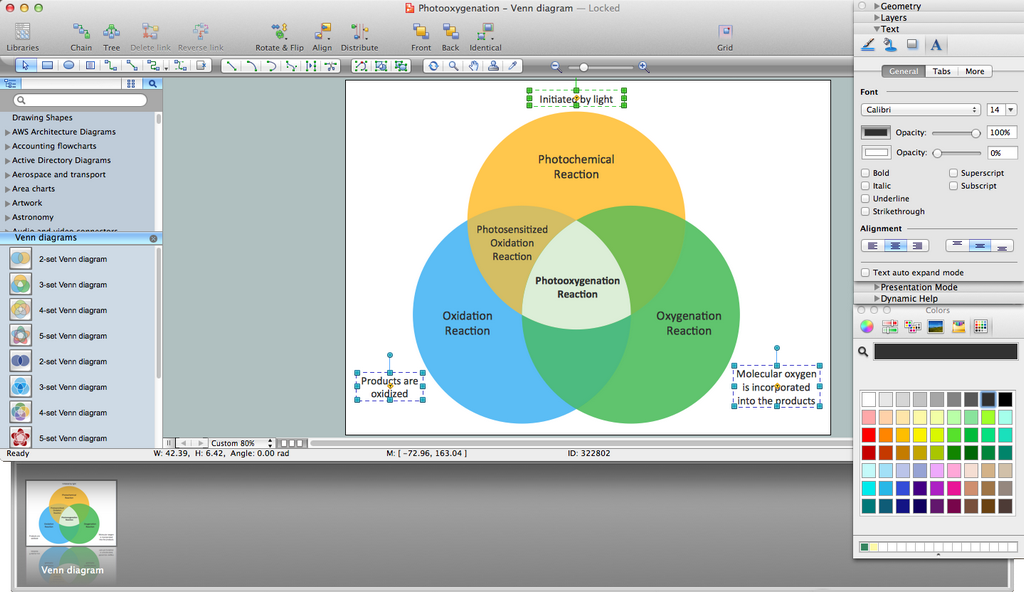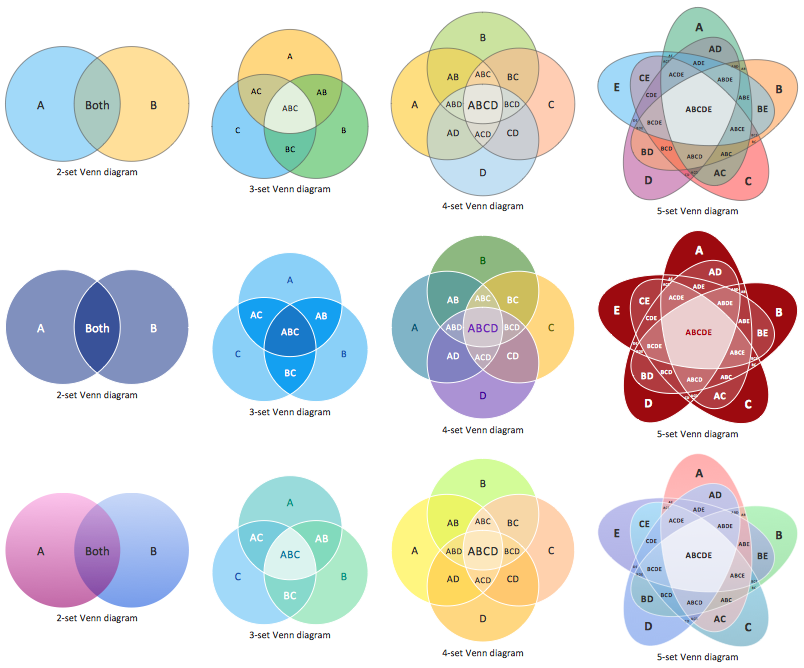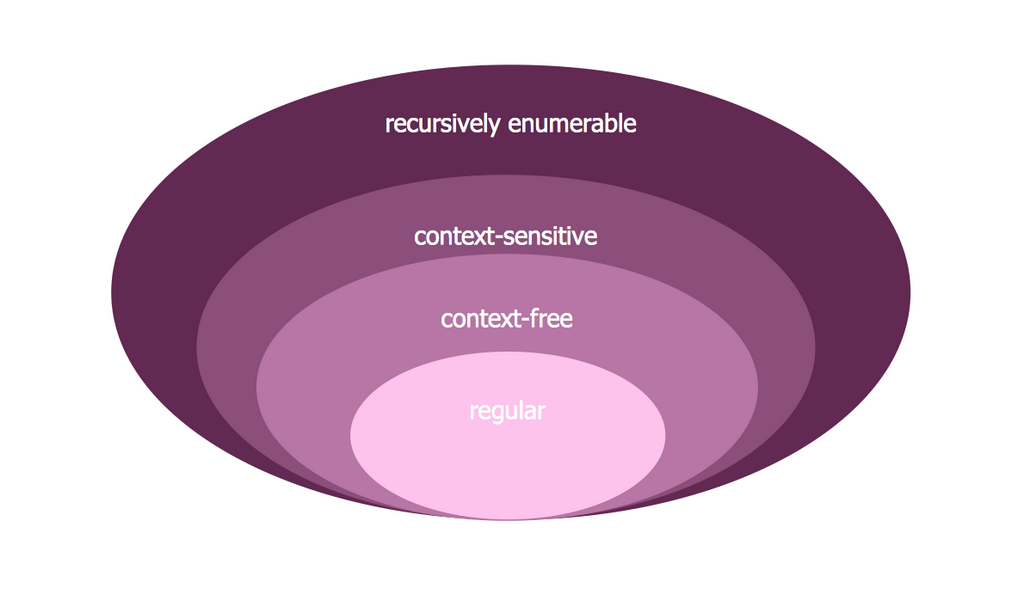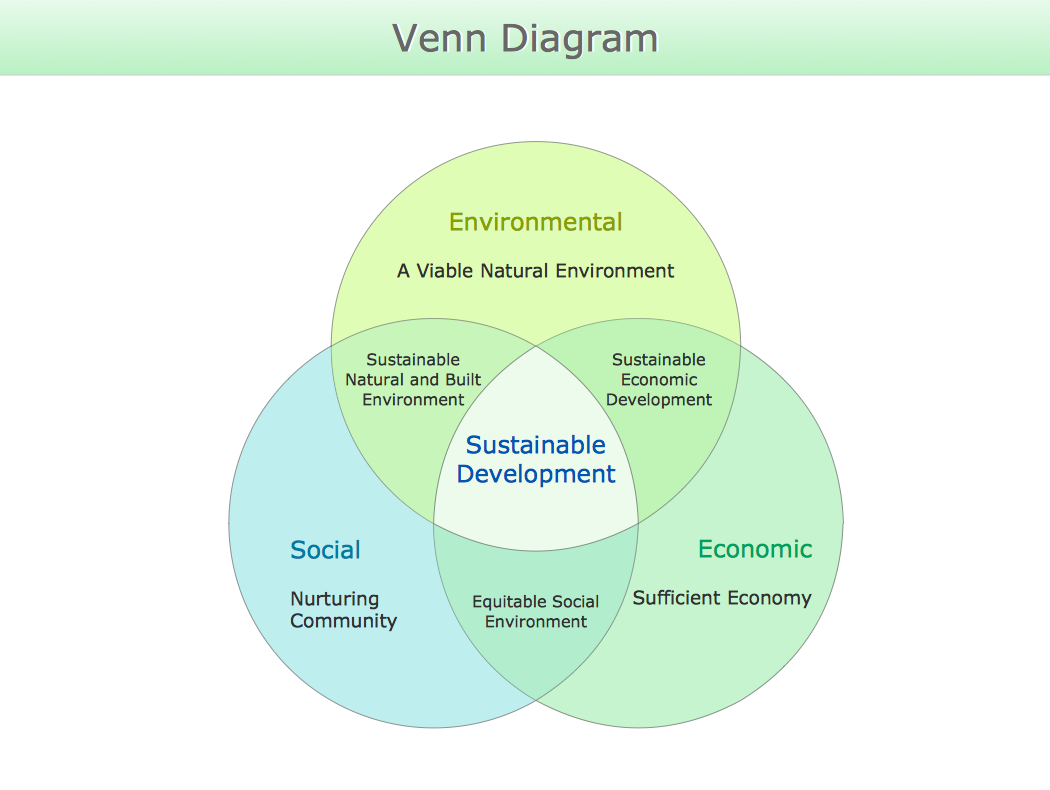# Venn Diagram Maker

ConceptDraw DIAGRAM diagramming and vector drawing software extended with Venn Diagrams solution from the "Diagrams" area of ConceptDraw Solution Park is a powerful Venn Diagram maker. Create fast and easy your own Venn Diagrams to visualize:

• All the possible logical relations between sets
• Simple set relationships in logic
• Statistics
• Mathematics
• Sociology
• Marketing
• Summarize the status and future viability of a project.Example 1. Venn Diagram Maker

Venn Diagrams Solution offers a set of predesigned Venn Diagram vector objects. Look at them - they are so varied. These are the objects for different number of sets and in various color gamuts, on any taste.Example 2. Venn Diagrams Library Design Elements

On the base of these predesigned objects was created collection of Venn Diagram examples and templates. It is included in Venn Diagrams solution and available from ConceptDraw STORE.Example 3. Venn Diagram Sample - Photooxygenation

The following features make ConceptDraw DIAGRAM the best Venn Diagram Maker:

1. You don't need to be an artist to draw professional looking diagrams in a few minutes.
2. Large quantity of ready-to-use vector objects makes your drawing diagrams quick and easy.
3. Great number of predesigned templates and samples give you the good start for your own diagrams.
4. ConceptDraw DIAGRAM provides you the possibility to use the grid, rules and guides. You can easily rotate, group, align, arrange the objects, use different fonts and colors to make your diagram exceptionally looking.
5. All ConceptDraw DIAGRAM documents are vector graphic files and are available for reviewing, modifying, and converting to a variety of formats: image, HTML, PDF file, MS PowerPoint Presentation, Adobe Flash, MS Visio.
6. Using ConceptDraw STORE you can navigate through ConceptDraw Solution Park, managing downloads and updates. You can access libraries, templates and samples directly from the ConceptDraw STORE.
7. If you have any questions, our free of charge support is always ready to come to your aid.

Related Solution:

### Hierarchical Network Topology

This sample was created in ConceptDraw DIAGRAM v12 diagramming and vector drawing software using the Computer and Networks solution from Computer and Networks area of ConceptDraw Solution Park. This sample shows the Hierarchical network topology. A Hierarchical network topology interconnects multiple groups that are located on the separate layers to form a larger network. Each layer concentrates on the specified functions, this allows to choose the right equipment for the layer.Picture: Hierarchical Network Topology
Related Solution:

### Venn Diagram Examples for Problem Solving. Computer Science. Chomsky Hierarchy

A Venn diagram, sometimes referred to as a set diagram, is a diagramming style used to show all the possible logical relations between a finite amount of sets. In mathematical terms, a set is a collection of distinct objects gathered together into a group, which can then itself be termed as a single object. Venn diagrams represent these objects on a page as circles or ellipses, and their placement in relation to each other describes the relationships between them. The Venn diagram example below visualizes the the class of language inclusions described by the Chomsky hierarchy.Picture: Venn Diagram Examples for Problem Solving. Computer Science. Chomsky Hierarchy
Related Solution:

### Venn Diagram

Venn diagrams are illustrations used in the branch of mathematics known as set theory. They show the mathematical or logical relationship between different groups of things (sets). A Venn diagram shows all the possible logical relations between the sets.Picture: Venn Diagram
Related Solution: